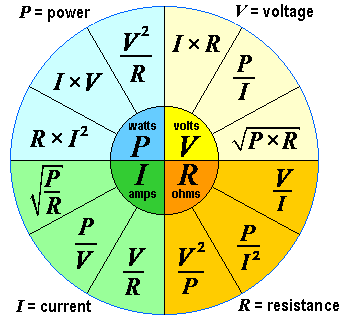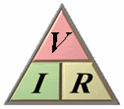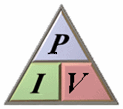Deutsche VersionThe Formula WheelFormulas of Electrical Engineering
for electric voltage V, current I, resistance R, impedance Z, power P
Magic circle − Formulas for calculating any combination of electrical units

 Principles of Electrical Engineering Physics Formulary and Electricity Equations Formula Wheel ▼ Important Formulas Electrical engineering laws Electronic engineering laws Electrical formulas as circle diagram (pie chart)V comes from "voltage" and E from "electromotive force emf". E means also energy, so let's choose V. Energy = voltage × charge. E = V × Q. Some like better to stick to E instead to V, so do it.

 Voltage V = I × R = P / I = √(P × R) in volts V          Current I = V / R = P / V = √(P / R) in amperes A Resistance R = V / I = P / I2 = V2/ P in ohms Ω      Power P = V × I = R × I2= V2/ R in watts W

Electric current, electric power, electricity − Formulas and calculations

Acoustics, sound, and vibrations − Acoustic equivalent for ohm's law

 In sound engineering there is no Power matching or Impedance matching. In audio we use only Voltage bridging or high Impedance bridging Zout < Zin. This includes also the connection from the power amplifier to the speaker.

Electric voltage V = I × R      (Ohm's law formula)
Electric voltage = amperage × resistance

Please enter two values, the third value will be calculated.

 Electric voltage V voltsAmperage I amps Resistance R ohms V = I × R              I = V ⁄ R              R = V ⁄ I
Electric power P = I × V      (Watt's power law formula)
Electric power = amperage × voltage

Please enter two values, the third value will be calculated.

 Electric Power P wattsAmperage I amps Voltage V volts P = I × V              I = P ⁄ V              V = P ⁄ I

If the unit of power P = I × V and of voltage V = I · R is needed
look for"The Big Power Formulas":
Calculations: Power (watt), voltage, current, and resistance